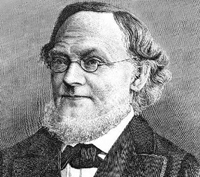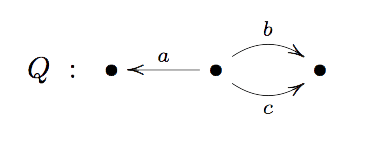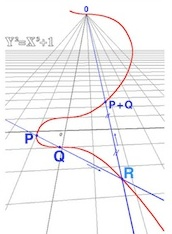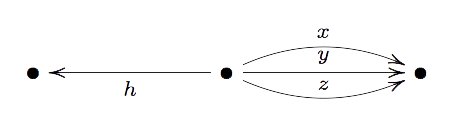A standard Grassmannian $Gr(m,V)$ is the manifold having as its points all possible $m$-dimensional subspaces of a given vectorspace $V$. As an example, $Gr(1,V)$ is the set of lines through the origin in $V$ and therefore is the projective space $\mathbb{P}(V)$. Grassmannians are among the nicest projective varieties, they are smooth and allow a cell decomposition.

A quiver $Q$ is just an oriented graph. Here’s an exampleA representation $V$ of a quiver assigns a vector-space to each vertex and a linear map between these vertex-spaces to every arrow. As an example, a representation $V$ of the quiver $Q$ consists of a triple of vector-spaces $(V_1,V_2,V_3)$ together with linear maps $f_a~:~V_2 \rightarrow V_1$ and $f_b,f_c~:~V_2 \rightarrow V_3$.

A sub-representation $W \subset V$ consists of subspaces of the vertex-spaces of $V$ and linear maps between them compatible with the maps of $V$. The dimension-vector of $W$ is the vector with components the dimensions of the vertex-spaces of $W$.

This means in the example that we require $f_a(W_2) \subset W_1$ and $f_b(W_2)$ and $f_c(W_2)$ to be subspaces of $W_3$. If the dimension of $W_i$ is $m_i$ then $m=(m_1,m_2,m_3)$ is the dimension vector of $W$.

The quiver-analogon of the Grassmannian $Gr(m,V)$ is the Quiver Grassmannian $QGr(m,V)$ where $V$ is a quiver-representation and $QGr(m,V)$ is the collection of all possible sub-representations $W \subset V$ with fixed dimension-vector $m$. One might expect these quiver Grassmannians to be rather nice projective varieties.

However, last week Markus Reineke posted a 2-page note on the arXiv proving that every projective variety is a quiver Grassmannian.Let’s illustrate the argument by finding a quiver Grassmannian $QGr(m,V)$ isomorphic to the elliptic curve in $\mathbb{P}^2$ with homogeneous equation $Y^2Z=X^3+Z^3$.

Consider the Veronese embedding $\mathbb{P}^2 \rightarrow \mathbb{P}^9$ obtained by sending a point $(x:y:z)$ to the point

$(x^3:x^2y:x^2z:xy^2:xyz:xz^2:y^3:y^2z:yz^2:z^3)$

The upshot being that the elliptic curve is now realized as the intersection of the image of $\mathbb{P}^2$ with the hyper-plane $\mathbb{V}(X_0-X_7+X_9)$ in the standard projective coordinates $(x_0:x_1:\cdots:x_9)$ for $\mathbb{P}^9$.

To describe the equations of the image of $\mathbb{P}^2$ in $\mathbb{P}^9$ consider the $6 \times 3$ matrix with the rows corresponding to $(x^2,xy,xz,y^2,yz,z^2)$ and the columns to $(x,y,z)$ and the entries being the multiplications, that is

$$\begin{bmatrix} x^3 & x^2y & x^2z \\ x^2y & xy^2 & xyz \\ x^2z & xyz & xz^2 \\ xy^2 & y^3 & y^2z \\ xyz & y^2z & yz^2 \\ xz^2 & yz^2 & z^3 \end{bmatrix} = \begin{bmatrix} x_0 & x_1 & x_2 \\ x_1 & x_3 & x_4 \\ x_2 & x_4 & x_5 \\ x_3 & x_6 & x_7 \\ x_4 & x_7 & x_8 \\ x_5 & x_8 & x_9 \end{bmatrix}$$

But then, a point $(x_0:x_1: \cdots : x_9)$ belongs to the image of $\mathbb{P}^2$ if (and only if) the matrix on the right-hand side has rank $1$ (that is, all its $2 \times 2$ minors vanish). Next, consider the quiverand consider the representation $V=(V_1,V_2,V_3)$ with vertex-spaces $V_1=\mathbb{C}$, $V_2 = \mathbb{C}^{10}$ and $V_2 = \mathbb{C}^6$. The linear maps $x,y$ and $z$ correspond to the columns of the matrix above, that is

$$(x_0,x_1,x_2,x_3,x_4,x_5,x_6,x_7,x_8,x_9) \begin{cases} \rightarrow^x~(x_0,x_1,x_2,x_3,x_4,x_5) \\ \rightarrow^y~(x_1,x_3,x_4,x_6,x_7,x_8) \\ \rightarrow^z~(x_2,x_4,x_5,x_7,x_8,x_9) \end{cases}$$

The linear map $h~:~\mathbb{C}^{10} \rightarrow \mathbb{C}$ encodes the equation of the hyper-plane, that is $h=x_0-x_7+x_9$.

Now consider the quiver Grassmannian $QGr(m,V)$ for the dimension vector $m=(0,1,1)$. A base-vector $p=(x_0,\cdots,x_9)$ of $W_2 = \mathbb{C}p$ of a subrepresentation $W=(0,W_2,W_3) \subset V$ must be such that $h(x)=0$, that is, $p$ determines a point of the hyper-plane.

Likewise the vectors $x(p),y(p)$ and $z(p)$ must all lie in the one-dimensional space $W_3 = \mathbb{C}$, that is, the right-hand side matrix above must have rank one and hence $p$ is a point in the image of $\mathbb{P}^2$ under the Veronese.

That is, $Gr(m,V)$ is isomorphic to the intersection of this image with the hyper-plane and hence is isomorphic to the elliptic curve.

The general case is similar as one can view any projective subvariety $X \rightarrow \mathbb{P}^n$ as isomorphic to the intersection of the image of a specific $d$-uple Veronese embedding $\mathbb{P}^n \rightarrow \mathbb{P}^N$ with a number of hyper-planes in $\mathbb{P}^N$.